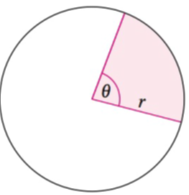Chapter 8.1, Problem 53E### Calculus: An Applied Approach (Min...

10th Edition
Ron Larson
ISBN: 9781305860919

#### Solutions

Chapter
Section### Calculus: An Applied Approach (Min...

10th Edition
Ron Larson
ISBN: 9781305860919
Textbook Problem
1 views

# Area of a Sector of a Circle In Exercises 53 and 54, use the following information. A sector of a circle is the region bounded by two radii of the circle and their intercepted arc (see figure).For a circle of radius r, the area A of a sector of the circle with central angle θ (in radians) is given by A = 1 2 r 2 θ .Sprinkler System A sprinkler in a garden is set to spray water over a distance of 25 feet and rotates through an angle of 120 ° . Find the area of the region.

To determine

To calculate: The area of the region if the water is sprayed from a sprinkler which rotates through an angle of 120°. It sprays water over a distance of 25 ft.

Explanation

Given Information:

The water is sprayed from a sprinkler which rotates through an angle of 120°. It sprays water over a distance of 25 ft. The area of a sector of a circle of radius r and central angle θ is A=12r2θ.

Formula used:

In order to convert the angle from degree to radian, multiply the angle by π radians180°.

Calculation:

Consider the angle of rotation be 120°.

Multiply angle 120° by π radians180° to find the angle in radians.

The sprinkler sprays water over a distance or radius of r=25 ft

### Still sussing out bartleby?

Check out a sample textbook solution.

See a sample solution

#### The Solution to Your Study Problems

Bartleby provides explanations to thousands of textbook problems written by our experts, many with advanced degrees!

Get Started

#### Divide: 4x212x6

Elementary Technical Mathematics

#### In Exercises 724, compute the indicated products. 

Finite Mathematics for the Managerial, Life, and Social Sciences

#### True or False: is a rational function.

Study Guide for Stewart's Single Variable Calculus: Early Transcendentals, 8th